# 12.E: Organic Reactions (Exercises)

$$\newcommand{\vecs}{\overset { \rightharpoonup} {\mathbf{#1}} }$$ $$\newcommand{\vecd}{\overset{-\!-\!\rightharpoonup}{\vphantom{a}\smash {#1}}}$$$$\newcommand{\id}{\mathrm{id}}$$ $$\newcommand{\Span}{\mathrm{span}}$$ $$\newcommand{\kernel}{\mathrm{null}\,}$$ $$\newcommand{\range}{\mathrm{range}\,}$$ $$\newcommand{\RealPart}{\mathrm{Re}}$$ $$\newcommand{\ImaginaryPart}{\mathrm{Im}}$$ $$\newcommand{\Argument}{\mathrm{Arg}}$$ $$\newcommand{\norm}{\| #1 \|}$$ $$\newcommand{\inner}{\langle #1, #2 \rangle}$$ $$\newcommand{\Span}{\mathrm{span}}$$ $$\newcommand{\id}{\mathrm{id}}$$ $$\newcommand{\Span}{\mathrm{span}}$$ $$\newcommand{\kernel}{\mathrm{null}\,}$$ $$\newcommand{\range}{\mathrm{range}\,}$$ $$\newcommand{\RealPart}{\mathrm{Re}}$$ $$\newcommand{\ImaginaryPart}{\mathrm{Im}}$$ $$\newcommand{\Argument}{\mathrm{Arg}}$$ $$\newcommand{\norm}{\| #1 \|}$$ $$\newcommand{\inner}{\langle #1, #2 \rangle}$$ $$\newcommand{\Span}{\mathrm{span}}$$

These are homework exercises to accompany Chapter 12 of the University of Kentucky's LibreText for CHE 103 - Chemistry for Allied Health. Answers are below the questions.

## Questions

### 12.1: Organic Reactions

Q12.1.1

Explain the difference between hydrolysis and condensation reactions.

Q12.1.2

What is being added in a hydrogenation reaction? Hydration? Bromination?

Q12.1.3

What is the product of the oxidation of a primary alcohol? A secondary alcohol?

Q12.1.4

Why is a condensation reaction also called a dehydration reaction?

Q12.1.5

Draw the structure for the primary product of each reaction..

1. CH3CH=CH2 + Br2
2. CH3CH2CH=CH2 + H2
3. CH3CH=CHCH3 + H2O →
4. CH3CH2CH=CH2 + H2O →
5. CH3CH2CH=CHCH3 + H2O →

Q12.1.6

Draw the structure of the compound that would be formed from the single oxidation of each compound.

 a.b.c.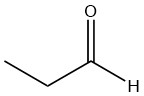Q12.1.7

Draw the structure of the compound that would be formed from the complete oxidation of each compound.

 a.b.c.Q12.1.8

Draw the structure of the compound that would be formed from a single reduction of each compound.

 a.b.c.Q12.1.9

Draw the product(s) for the hydrolysis of the given reactant. The red bond in the reactant is the one that is broken.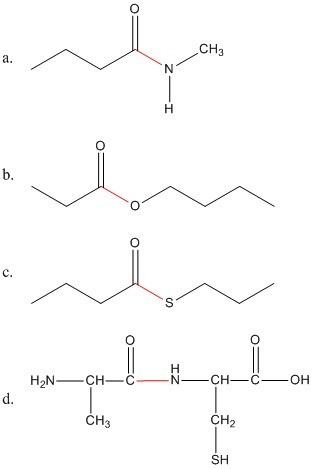Q12.1.10

Draw the product(s) for the condensation of the given reactants. The layout of the molecules indicates where the bond will form between them. If there are three molecules, two condensations will occur to produce one final product.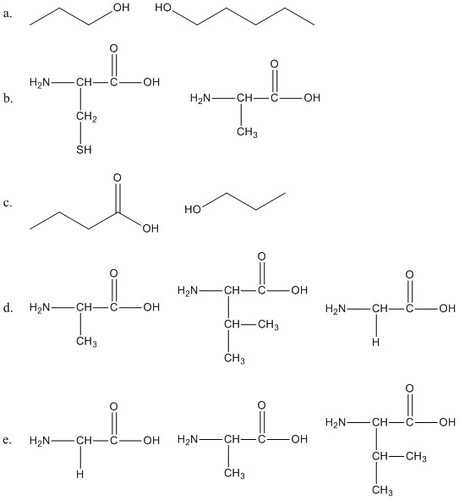Q12.1.11

Compare the reactants and products in part d and e of 12.1.10. Are the reactants the same or different? Are the products the same or different? Why?

### 12.1: Organic Reactions

Q12.1.1

Hydrolysis is a molecule breaking apart after the addition of water. Condensation is two molecules coming together and producing H2O.

Q12.1.2

Q12.1.3

A primary alcohol oxidizes to an aldehyde and then to a carboxylic acid. A secondary alcohol oxidizes to a ketone.

Q12.1.4

Because water is released during a condensation reaction.

Q12.1.5

 a.Each bromine atom is added to one of the carbons in the double bond. b. CH3CH2CH2CH3 Each hydrogen atom is added to one of the carbons in the double bonds. c.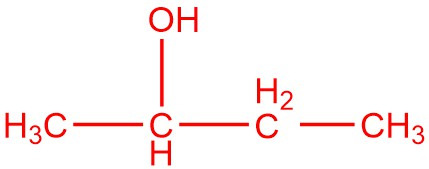Water is added as H and OH. The carbons in the double bond each have the same number of hydrogen atoms so Markovnikov's rule does not apply. The molecule is symmetrical so adding the OH group to either carbon results in the same molecule. d.Water is added as H and OH. The carbon atoms in the double bond have different number of hydrogen atoms so Markovnikov's rule does apply. The H is added to the carbon with more hydrogen atoms and the OH is added to the carbon with fewer hydrogen atoms. e.andWater is added as H and OH. The carbon atoms in the double bond have the same number of hydrogen atoms on each so Markovnikov's rule does not apply. There are two possible products because adding the OH to the carbon on the left side of the double bond results in a different molecule than adding the OH to the carbon on the right side of the double bond. This differs from c because the molecule is not symmetrical.

Q12.1.6

 a.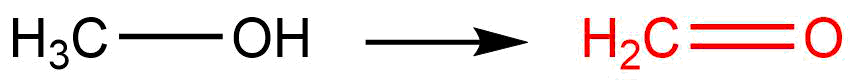b.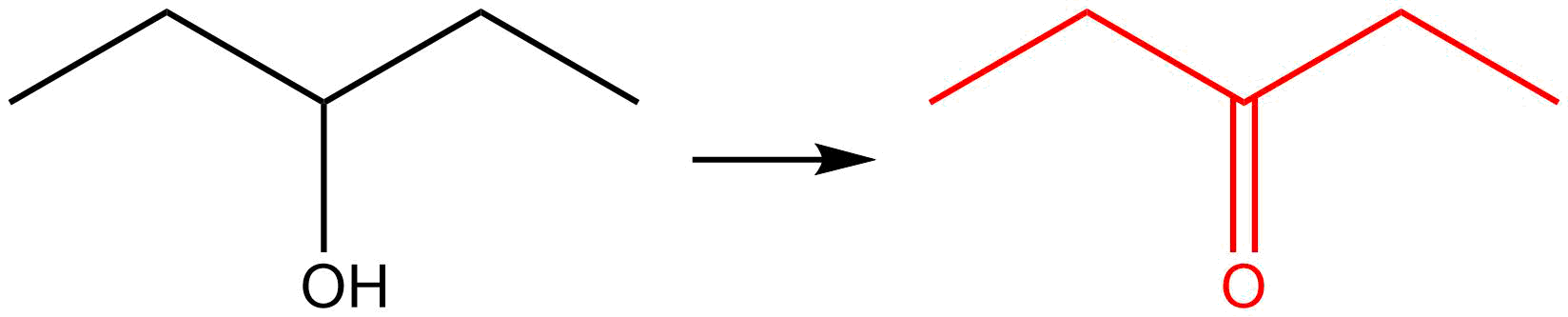c.Q12.1.7

 a.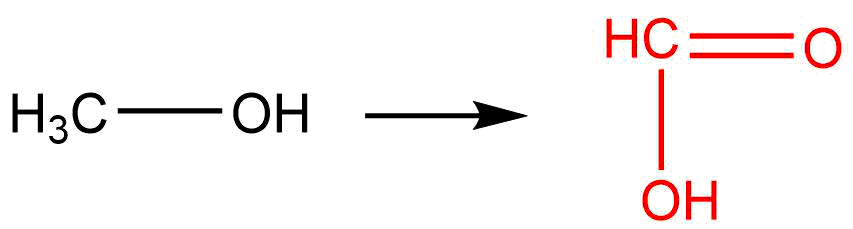b.c.Q12.1.8

 a.b.c.Q12.1.9

 a.b.c.d.Q12.1.10

 a.b.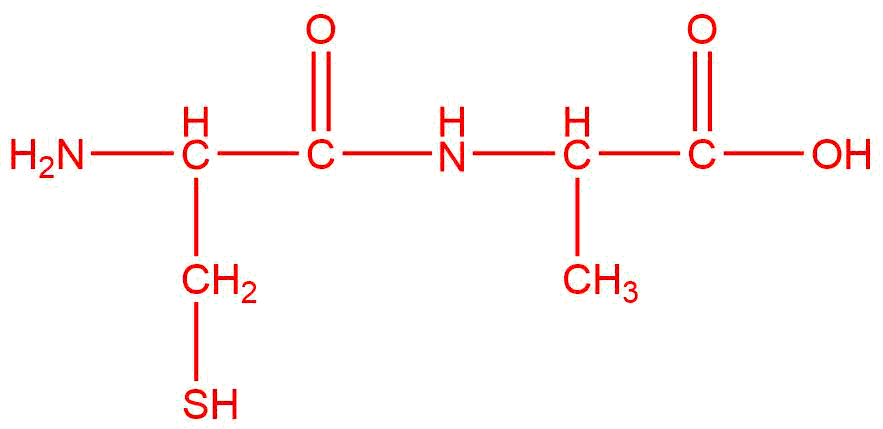c.d.e.Q12.1.11

The reactants are the same, but the products are different because they are combined in a different order.

This page titled 12.E: Organic Reactions (Exercises) is shared under a CK-12 license and was authored, remixed, and/or curated by CK-12 Foundation.